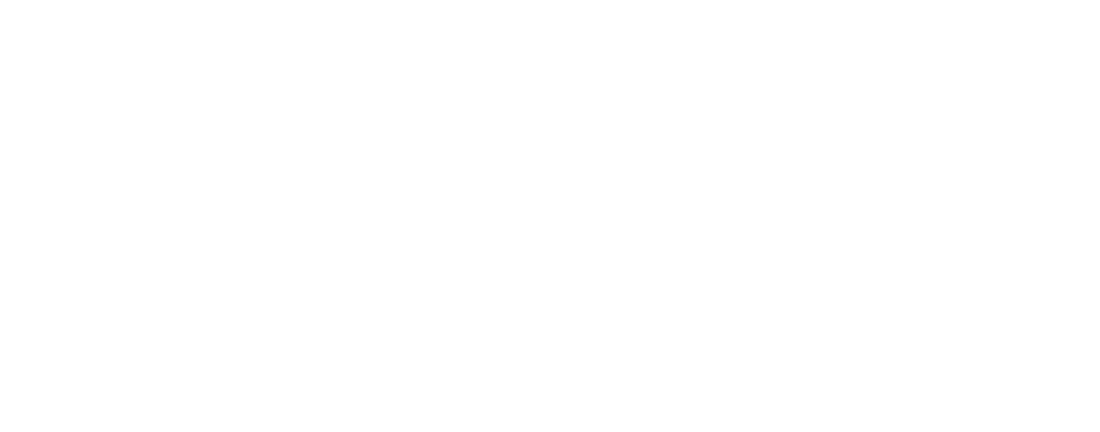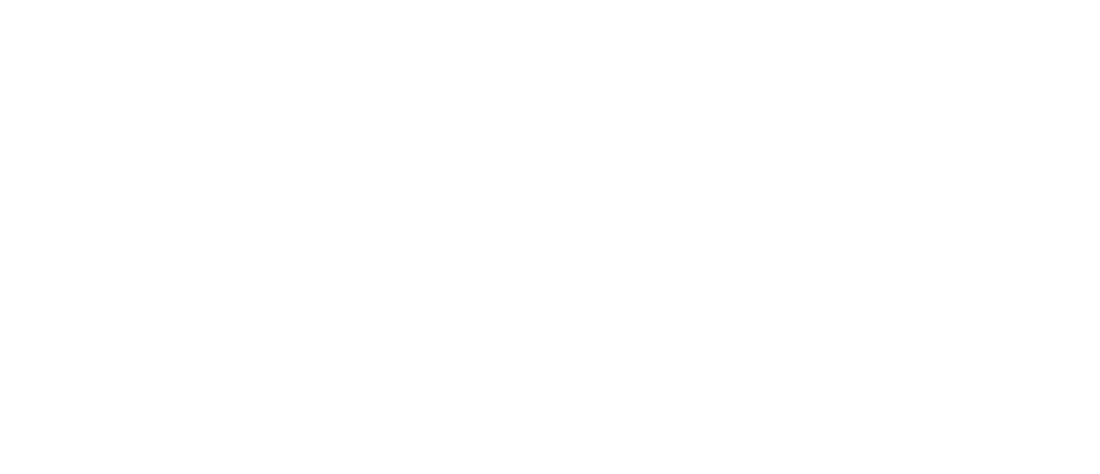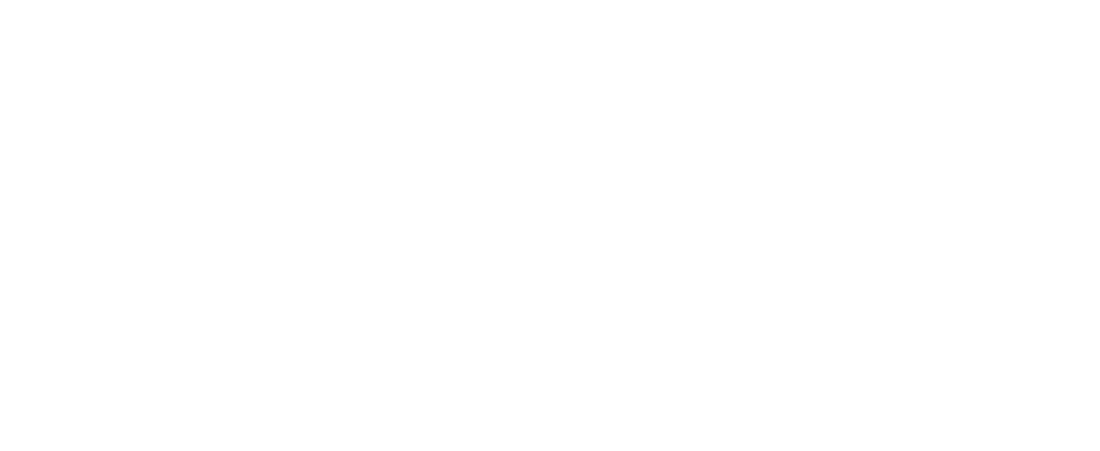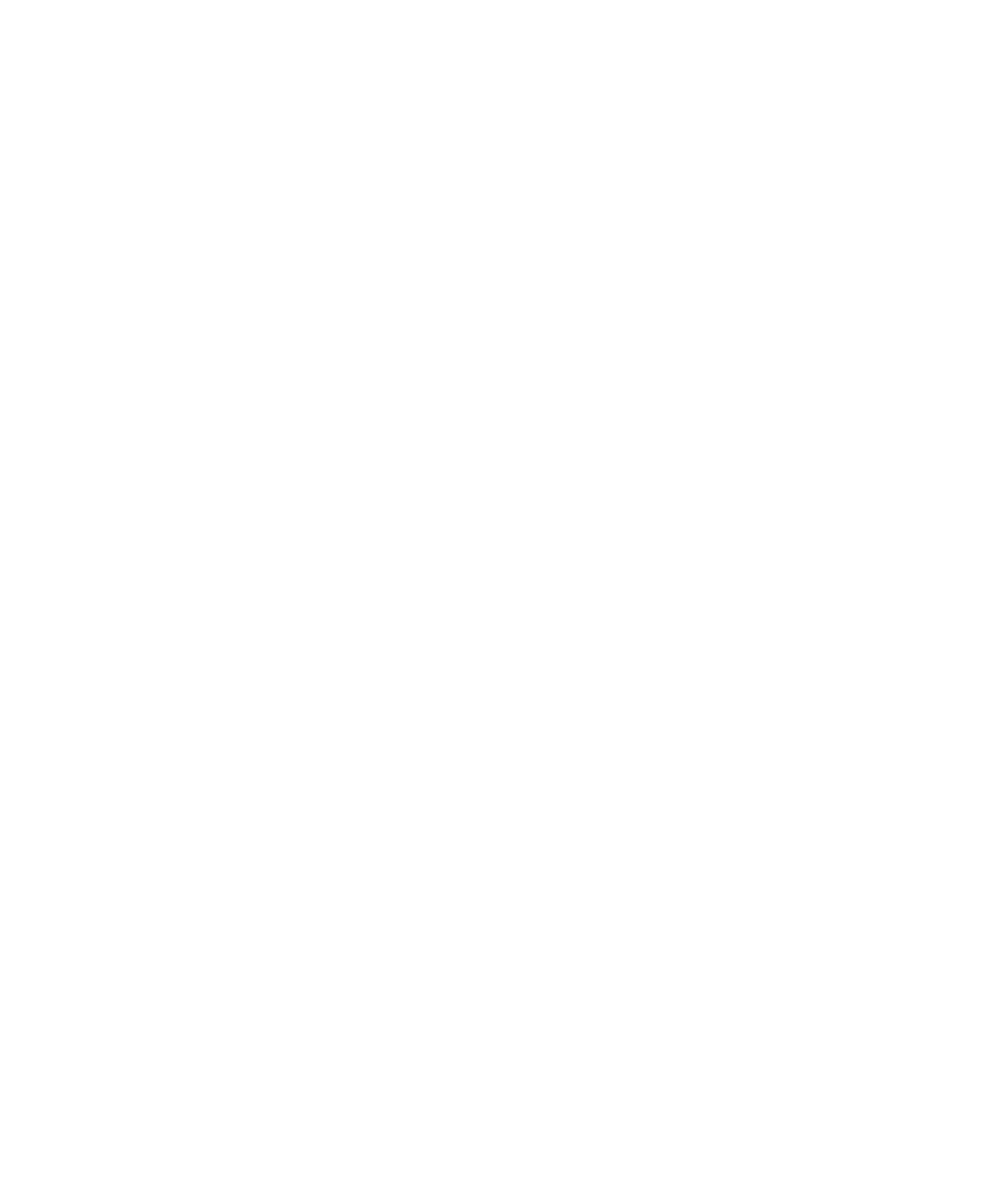# 1.1.2 Magnification Calculations

### Magnification Calculations

• Magnification is how many times bigger the image of a specimen observed is in comparison to the actual (real-life) size of the specimen
• The magnification (M) of an object can be calculated if both the size of the image (I), and the actual size of the specimen (A), is knownAn equation triangle for calculating magnification

#### Worked example: Magnification

An image of an animal cell is 30 mm in size and it has been magnified by a factor of X 3000.
What is the actual size of the cell?

To find the actual size of the cell:Worked example using the equation triangle for magnification

• The size of cells is typically measured using the micrometre (μm) scale, with cellular structures measured in either micrometers (μm) or nanometers (nm)
• When doing calculations all measurements must be in the same units. It is best to use the smallest unit of measurement shown in the question
• To convert units, multiply or divide depending if the units are increasing or decreasing
• Magnification does not have unitsConverting units of measurement

• There are 1000 nanometers (nm) in a micrometre (µm)
• There are 1000 micrometres (µm) in a millimetre (mm)
• There are 1000 millimetres (mm) in a metre (m)

#### Worked example: Magnification drawingCheck that units in magnification questions are the same

• Remember that 1mm = 1000µm
• 2000 / 1000 = 2, so the actual thickness of the leaf is 2 mm and the drawing thickness is 50 mm
• Magnification = image size / actual size = 50 / 2 = 25
• So the magnification is x 25### Author: Jenna

Jenna studied at Cardiff University before training to become a science teacher at the University of Bath specialising in Biology (although she loves teaching all three sciences at GCSE level!). Teaching is her passion, and with 10 years experience teaching across a wide range of specifications – from GCSE and A Level Biology in the UK to IGCSE and IB Biology internationally – she knows what is required to pass those Biology exams.
CloseClose